# Finite State Machine (Finite Automata)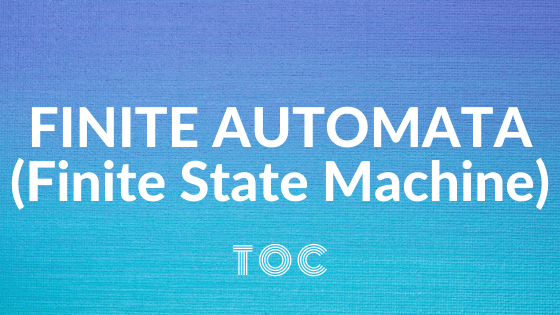## Finite Automata (Finite State Machine)

Finite State Machine is popularly referred to as Finite Automata. it’s the best model of computation and has very limited memory.

On the basis of output, we can differentiate in two parts: the first one is Finite Automata with output and another one is Finite Automata without output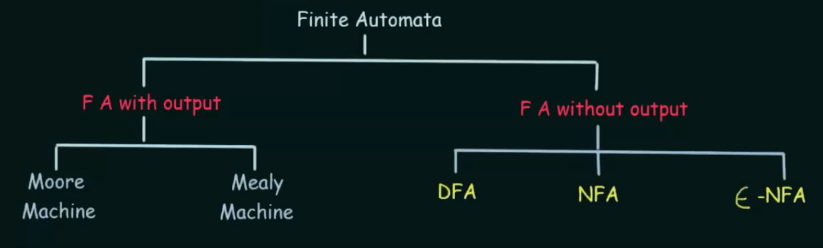## A FINITE AUTOMATON (FINITE AUTOMATA)

A finite automaton may be a five-tuple  (Q, Σ, δ, qº, F), where

1. Q is finite set called the states,
2. ∑ is finite set called the alphabet,
3. δ : Q X Σ → Q is that the transition function
4. q° ∈ Q is that the start state, and
5. F ⊆ Q is that the set of accept states.

The machine always recognizes only one language but it can accept many strings. The machine recognizes a language even when it is not accepting a string, which is known as the empty language ø.

## Deterministic Finite Automata (DFA)

• DFA stands for Deterministic Finite Automata.
• Deterministic shows the individuality of computation.
• The finite automata are referred to as deterministic finite automata if the machine is read an input string one symbol at a time.
• From the present state to subsequent state there’s just one path for specific input in DFA
• In Lexical Analysis ( in Compiler ) DFA contain multiple final states.

## Formal Definition of a Deterministic Finite Automata (DFA)

A DFA are often represented by a five-tuple (Q, ∑, δ, q0, F) where —

• Q = set of all finite set
• Σ = finite set of symbols referred to as the alphabet (a,b)
• δ = transition function i.e; what’s output, where δ: Q × ∑ → Q
• q0 = start state is additionally referred to as the initial state (q0 ∈ Q).
• F = set of ultimate states/state of Q (F ⊆ Q).

## Graphical Representation of DFA

A DFA even be represented by digraphs called a state diagram

• The state is represented by vertices.
• The arc labeled with an input character shows the transitions.
• An arrow (→) sigh of initial state .
• The final state is marked by a double circle.

## Deterministic Finite Automata (Example-1)

= {0, 00, 01, 000, 010,…….}

Let’s make a state diagram for this problem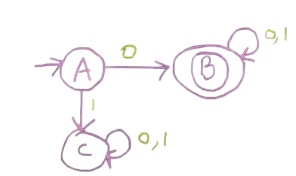Here

• A is known as an initial state
• B is known as the final state, and
• C is known as dead or trap state

Let’s make a string 001 using the above state diagram## Example 2

Q = {q0, q1, q2}

∑ = {01}

q0 = {q0}

F = {q2}

Transition Diagram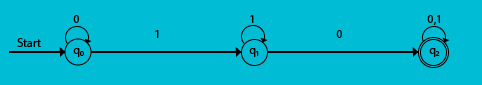Transition Table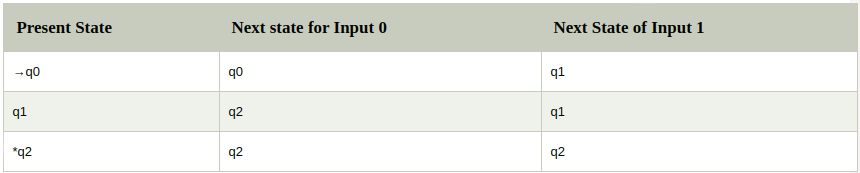## NONDETERMINISM

Nondeterminism may be a useful concept that has had an excellent impact on the idea of computation. In DFA when the machine is during a given state and reads subsequent input symbol, we all know what subsequent state are going to be – it’s determined. We call this deterministic computation.

Nondeterminism is a generalization of determinism, so every deterministic finite automaton (DFA) is an automatically nondeterministic finite automaton (NFA/NDFA)

## Non-Deterministic Finite Automata (NDFA/NFA)

• For NFA, any set Q we write P(Q) to be the collection of all subsets of Q.
• P(Q) =  power set of Q
• For any alphabet Σ, we Σε write to be Σ ∪ {ε}.
• NFA as δ: Q X Σε → P(Q) commonly written as δ: Q × ∑ → 2Q

#### Formal Definition of an NDFA/NFA

A NDFA is a 5-tuple (Q, ∑, δ, q0, F) where —

• Q = set of all finite set
• Σ = finite set of symbols known as the alphabet (a,b)
• δ = transition function i.e; what is output, where δ: Q × ∑ε → P(Q) or δ: Q × ∑ → 2Q
• q0 = start state is also known as the initial state (q0 ∈ Q).
• F = set of final states/state of Q (F ⊆ Q, set of accepting states).

## How does an NFA compute?

The root of the tree corresponds to the start of the computation at which the machine has multiple choices. as shown in below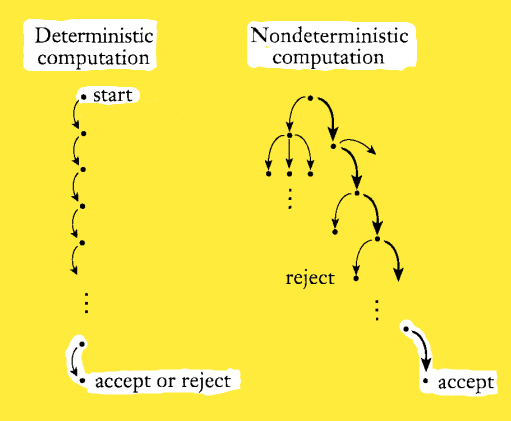## DFA vs NDFA

DFA NDFA
In DFA transition, we know the next state will be—it is determined. Therefore; it is known as deterministic In NDFA transition, the next states can be multiple for each input symbol. Therefore; it is known as non-deterministic
Every deterministic finite automaton is automatically a nondeterministic finite automaton Every nondeterministic finite automaton is not a deterministic finite automaton
Empty string transitions are not seen in DFA. Empty string permited in NFA
Backtracking is possible Backtracking is not always allowed.
Need more space. Need less space.
string  accepted: final state transits also. string  accepted: In a final state, At least one of all possible transitions ends